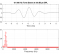## Compute inverse FIRStarted by 2 years ago6 replieslatest reply 2 years ago223 views
Dear DSP experts,

I have a measured FRF by impact testing and I have computed the IRF by ifft(). Now I want to compute the inverse IRF. I have read many posts in this forum about inverting FIR filters. As far as I understood, I must check where are my poles and zeros to see if my inverted FIR filter will be causal and stable. My initial idea was just inverting my FRF and computing again the corresponding IRF (inverse) by ifft(). Then, my poles become zeros and vice versa. When I do this I see that the inverse looks non-causal and quite noisy. Therefore, I want to check the zeros and poles. Here comes my first question:

1. How do you compute the zplot in Matlab from an FIR filter coming from a measurement?

My second question:

My third question, related to the second question:

3. Somewhere else I read that my inverted FIR might become an IIR. Is that true? And Why? Maybe that's why my inverted FIR becomes "longer".

I know there are other methods to compute the inverse FIR, (e.g. LMS inverse), but I want to try this method first.

[ - ]What are the terms FRF and IRF?

[ - ]FRF = Frequency Response Function

IRF = Impulse Response Function

FIR = Finite Impulse Response

[ - ]what is FRF, IRF and inverted IRF. OK we know what is iFFT.

What is inverted FIR filter, I assume you can reverse passband gain but any stopband info is lost for ever.

[ - ][ - ]If by inverting FIR you mean low to high pass (...etc) then you can do that in two ways at least:

1) flip spectrum then do ifft

2) multiply your LPF by (+1,-1,+1,-1...) i.e. invert sign of every other coefficient.

[ - ]